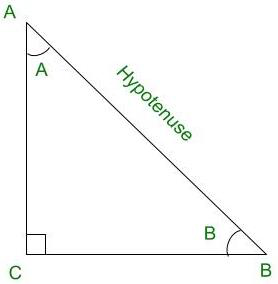# Given sin A = 12/37, find cos A and tan A

• Last Updated : 03 Sep, 2021

Trigonometry is the relation between the angles and sides of a right-angled triangle. In a right angles triangle, there are 3 angles of which one angle is a right angle (90°) and the other two angles are acute angles and there are 3 sides. The side opposite to the right angle is called Hypotenuse. There are 6 ratios between these sides based on the angle between them and they are called Trigonometric Ratios.

The 6 Trigonometric ratios are:

• Sine (sin)
• Cosine (cos)
• Tangent (tan)
• Cosecant (cosec)
• Secant (sec)
• Cotangent (cot)Right Angled Triangle ACB

Sine (sin):

Sine of an angle is defined by the ratio of lengths of sides which is opposite to the angle and the hypotenuse. For the above triangle, sin A = BC/AB

Cosine (cos):

Cosine of an angle is defined by the ratio of lengths of sides which is adjacent to the angle and the hypotenuse. For the above triangle, cos A = AC/AB

Tangent (tan):

Tangent of an angle is defined by the ratio of the length of sides which is opposite to the angle and the side which is adjacent to the angle. For the above triangle, tan A = BC/AC

Cosecant (cosec):

Cosecant of an angle is defined by the ratio of the length of the hypotenuse and the side opposite the angle. For the above triangle, cosec A = AB/BC

Secant (sec):

Secant of an angle is defined by the ratio of length of the hypotenuse and the side and the side adjacent to the angle For the above triangle, sec A = AB/AC

Cotangent  (cot):

Cotangent  of an angle is defined by the ratio of length of sides which is adjacent to the angle and the side which is opposite to the angle. For the above triangle, cot A = AC/BC

### Given sin A = 12/37, find cos A and tan A.

Solution:

It is given that,

sinA = 12/37                                     ………………….( 1 )

We know that, cos2X = 1 – sin2X

⇒ cos2A = 1 – sin2A

⇒ cos2A = 1 – (12 / 37)2

⇒ cos2A = 1 – (144 / 1369)

⇒ cos2A = (1369 – 144) / 1369

⇒ cos2A = 1225 /1369

⇒ cosA = √1225 / √1369

⇒ cosA = 35 / 37                              ………………….( 2 )

tanA = sinA/cosA

From (1) and (2),

⇒ tanA = (12/37) / (35/37)

⇒ tanA = 12/35

Therefore, the values of cosA and tanA are (35/37) and (12/35) respectively.

### Similar Problems

Question 1: If sinA = 3/5, find cosA and tanA

Solution:

It is given that,

sinA = 3 / 5   ⇢  (1)

We know that, cos2X = 1 – sin2X

⇒ cos2A = 1 – sin2A

⇒ cos2A = 1 – (3/5)2

⇒ cos2A = 1 – (9 / 25)

⇒ cos2A = (25 – 9) / 25

⇒ cos2A = 16 / 25

⇒ cosA = √16 / √25

⇒ cosA = 4 / 5   ⇢  (2)

tanA = sinA / cosA

From (1) and (2),

⇒ tanA = (3/5) / (4/5)

⇒ tanA = 3 / 4

Therefore, the values of cosA and tanA are (4/5) and (3/4) respectively.

Question 2: If Sin A = 3/4, Calculate cos A and tan A.

Solution:

It is given that,

sinA = 3 / 4    ⇢  (1)

We know that, cos2X = 1 – sin2X

⇒ cos2A = 1 – sin2A

⇒ cos2A = 1 – (3/4)2

⇒ cos2A = 1 – (9/16)

⇒ cos2A = (16 – 9)/16

⇒ cos2A = 7/16

⇒ cosA = √7/√16

⇒ cosA = √7/4    ⇢  (2)

tanA = sinA/cosA

From (1) and (2),

⇒ tanA = (3/4)/(√7/4)

⇒ tanA = 3/√7

Therefore, the values of cosA and tanA are (√7/4) and (3/√7) respectively.

Question 3: If cosA = 12/13, find sinA and tanA.

Solution:

It is given that,

cosA = 12 / 13    ⇢  (1)

We know that, sin2X = 1 – cos2X

⇒ sin2A = 1 – cos2A

⇒ sin2A = 1 – (12/13)2

⇒ sin2A = 1 – (144 / 169)

⇒ sin2A = (169 – 144) / 16

⇒ sin2A = 25 / 169

⇒ sinA = √25 / √169

⇒ sinA = 5 / 13    ⇢  (2)

tanA = sinA / cosA

From (1) and (2),

⇒ tanA = (5/13) / (12/13)

⇒ tanA = 5/12

Therefore, the values of sinA and tanA are (5/13) and (5/3) respectively.

My Personal Notes arrow_drop_up